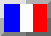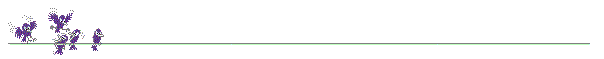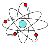Version francaise

## The electron-volt (unit of energy)

In particle physics, a unit of energy is commonly used: the electron-volt (eV).

1 eV = 1.6 10-19 Joules

• A 60 Watts electric lamp uses 60 Joules per second to give some light to you.
• 1 GeV = 1 billion of electron-volts
• A flying mosquito (0.01 g) is composed of about 3 1019 atoms and, to climb one meter, it needs about 0.0001 Joule, that is about 30 millionth of electron-volt per atoms of mosquito.Moreover, particles masses are expressed in a more convenient way thanks to the electron-volt.
The famous equation E = mc2 gives the following relationship:

1 eV/c2 = 1.8 10-36 kg

An electron, for instance, has a mass of 511 keV, that is about 10-30 kg.

Back to main page

Last update: 26/06/1999 : http://wwwlapp.in2p3.fr/neutrinos/agev.htmlDidier Verkindt